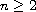Electron. J. Diff. Equ., Vol. 2015 (2015), No. 189, pp. 1-17.

### An Abel type cubic system Gary R. Nicklason

Abstract:
We consider center conditions for plane polynomial systems of Abel type consisting of a linear center perturbed by the sum of 2 homogeneous polynomials of degrees n and 2n-1 where. Using properties of Abel equations we obtain two general systems valid for arbitrary values on n. For the cubic n=2 systems we find several sets of new center conditions, some of which show that the results in a paper by Hill, Lloyd and Pearson which were conjectured to be complete are in fact not complete. We also present a particular system which appears to be a counterexample to a conjecture by Zoladek et al. regarding rational reversibility in cubic polynomial systems.

Submitted March 30, 2015. Published July 16, 2015.
Math Subject Classifications: 34A05, 34C25.
Key Words: Center-focus problem; Abel differential equation; constant invariant; symmetric centers.

Show me the PDF file (258 KB), TEX file, and other files for this article.Gary R. Nicklason Mathematics, Physics and Geology Cape Breton University Sydney, Nova Scotia, B1P 6L2, Canada email: gary_nicklason@cbu.ca